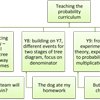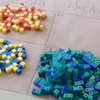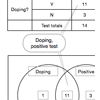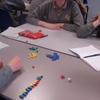# Probability Articles### Probability Through Problems: a new approach to teaching probability

##### Age 11 to 16### Introducing Probability

##### Age 11 to 16##### Age 11 to 14

Why the tree diagram is fundamental to studying probability - and why it should be introduced right from the start.### Probability Calculations from Tree Diagrams

##### Age 11 to 16

Progression from natural frequencies to proportions to the multiplication rule, and hence to probability trees.### Learning Probability Through Mathematical Modelling

##### Age 11 to 16

Moving from the particular to the general, then revisiting the particular in that light, and so generalising further.### Tree Diagrams, 2-way Tables and Venn Diagrams

##### Age 11 to 18

Representing frequencies and probabilities diagrammatically, and using the diagrams as interpretive tools.### Independence and Dependence

##### Age 11 to 18

When are events independent of each other? Sampling with and without replacement.### Conditional Probability Is Important for All Students!

##### Age 11 to 18

Understanding statistics about testing for cancer or the chance that two babies in a family could die of SIDS is a crucial skill for ALL students.